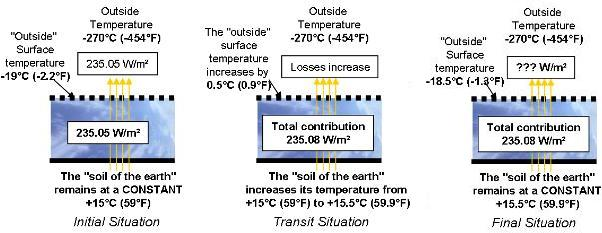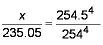Chargement...

# 5) Let’s assume global warming is observed from space 5) Let’s assume global warming is observed from space

5) Let’s assume global warming is observed from space

Let’s first assume that Global Warming is “observed” from space and that the increase of 0.5 [°C] (0.9 °F) at the surface is integrally sent back towards "the periphery of the planet" (which assumes that the thermal resistance of the atmosphere did not vary).Let’s compute under these conditions the additional power that would be rejected towards space due to the increase in temperature difference between the “exterior surface” of the planet (increase between -19 and -18.5 [°C] (-2.2 and - 1.3°F) and the stable universe (at -270 [°C], -454°F).

## Question

• Given that the flux exchanged between the planet and space was 235.05 [W/m² ] prior to Global Warming, under a temperature of the planet‘s “exterior surface” of -19 [°C] (-2.2 °F) and a temperature of the universe of -270 [°C] (-454°F).
• Given that the flux exchanged by radiation is of the following type:

Flux = k x (T14 - T24
With:
Flux: power exchanged by radiation in [W]
T1    : temperature of the warmer element in [K]
T2    : temperature of the colder element in [K]
k     : constant ratio in [W/K4]

Given that the surface of the earth amounts to 5.1 x E14 [m² ]
Determine the additional thermal power that would be evacuated from the planet if its “exterior surface” temperature changed from -19 to -18.5 [°C] (from -2.2°F to -1.3°F).

9.5 x E14[W] , which is 63 times more than the additional power of 1.5 x E13 [W]  which the planet must evacuate because of our human activity.

Explanation:
Flux = k x (T14 – T24
With T1 and T2 expressed in Kelvin (see file Températures, dilatation en génie climatique)
T1 = -19 [°C] (-2.2°F) expressed in Kelvin,
T1 = -19 + 273 = 254 [K]
T2 = -18.5 [°C] (-1.3°F) expressed in Kelvin,
T2 = -18.5 + 273 = 254.5 [K]
Let X be the flux we are after (in [W/m²])
We can write:
235.05 = k x (2544 - 34)
X = k x (254.54 - 34)

Ignoring the 34 term we can write:Hence X = 236.9 [W/m² ]
Hence for the planet whose surface is 5.1 x E14 [m² ], an increase in the dissipation towards space of:
(236.9 - 235.05) x  5.1 x E14= 9.5 x  E14 [W]

Which is 63 times more than the additional power of 1.5 x E13 [W] which the planet must evacuate because of our human activity.

If the current Global Warming is “observed” from space, the additional power evacuated is over 60 times greater than the one corresponding to human activity.

Under these conditions, the observed increase in temperature would not only be due to the energy we consume but also to a variation of the absorbed solar power. This increase would correspond to a power that would be too large to be explained only by a variation of solar activity. It would therefore suppose a slight modification of the absorption coefficient of the planet.

This scenario would be the most worrisome because the “heating reserve” of the sun is incomparable to what we can and will be able to emit as part of human activity.